# How to Backdoor Diffie-Hellman - Cryptology ePrint Archive

## Transcription

How to Backdoor Diffie-Hellman - Cryptology ePrint Archive
```How to Backdoor Diffie-Hellman
David Wong
NCC Group, June 2016
1
Abstract
Introduction
Around Christmas 2015 Juniper, a networking
hardware company, released an out-of-cycle security bulletin1 . Two vulnerabilities were disclosed without much details to help us grasp
the seriousness of the situation. Fortunately,
at this period of the year many researchers
were home with nothing else to do but to solve
this puzzle. By quickly comparing both the
patched and vulnerable binaries, the two issues were pinpointed. While one of the vulnerabilities was a simple “master”-password implemented at a crucial step of the product’s
authentication, the other discovery was a bit
more subtle: a unique value was modified.
More accurately, a number in the source code
was replaced. The introduction of the vulnerability was so simple, and due to the fact that
the number was stored as a string of hexadecimal digits, the trivial use of the UNIX command line tool strings was enough to discover
it.
The special value ended up being a constant
used in the system’s pseudo-random number
generator (PRNG) Dual EC, an odd algorithm believed to have been backdoored by the
NSA2 . The PRNG’s core has the ability to provide a Nobody-But-Us (NOBUS) trapdoor: a
Lately, several backdoors in cryptographic
constructions, protocols and implementations
have been surfacing in the wild: Dual-EC in
RSA’s B-Safe product, a modified Dual-EC
in Juniper’s operating system ScreenOS and
a non-prime modulus in the open-source tool
socat. Many papers have already discussed
the fragility of cryptographic constructions
not using nothing-up-my-sleeve numbers, as
well as how such numbers can be safely picked.
However, the question of how to introduce a
backdoor in an already secure, safe and easy
to audit implementation has so far rarely been
researched (in the public).
We present two ways of building a NobodyBut-Us (NOBUS) Diffie-Hellman backdoor: a
composite modulus with a hidden subgroup
(CMHS) and a composite modulus with a
smooth order (CMSO). We then explain how
we were able to subtly implement and exploit
it in a local copy of an open source library
using the TLS protocol.
Keywords:
Diffie-Hellman, Ephemeral,
DHE, NOBUS, Backdoor, Discrete Logarithm,
Small Subgroup Attack, Pohlig-Hellman, Pollard Rho, Factorization, Pollard’s p-1, ECM,
Dual-EC, Juniper, socat
1
https://kb.juniper.net/InfoCenter/index?
page=content&id=JSA10713
2
Daniel J. Bernstein, Tanja Lange, and Ruben
Niederhagen. Dual EC: A Standardized Back Door.
Cryptology ePrint Archive, Report 2015/767. http :
//eprint.iacr.org/2015/767. 2015
1
one more time, magically allowing another organization, or person, to access that backdoor
in place of the NSA or Juniper.
Although the quest to find Juniper’s backdoor
and the numerous open questions that arose
from that work is a fascinating read by
itself4 , it is only the introduction of the work
you are currently reading. Here we aim to
show how secure and strong cryptographic
constructions are a single and subtle change
away from being your own secretive peep show.
Figure 1: The strings of the patched binary
On February 1st, 2016, only a few months after
Juniper’s debacle, socat published a security
advisory of its own5 :
In the OpenSSL address implementation the hard coded 1024-bit DH p
parameter was not prime. The effective cryptographic strength of a key
exchange using these parameters was
weaker than the one one could get
by using a prime p. Moreover, since
there is no indication of how these parameters were chosen, the existence
of a trapdoor that makes possible for
an eavesdropper to recover the shared
secret from a key exchange that uses
them cannot be ruled out.
Figure 2: The strings of the vulnerable binary
secret passage that can only be accessed by the
people holding the secret key. In our case: the
elliptic curve discrete logarithm k in the Dual
EC equation Q = [k]P (where P and Q are
the two elliptic curve points used in the foundation of Dual EC).
Solely the NSA is thought to be in possession
of that k value, making them the only ones
able to climb back to the PRNG’s internal
state from random outputs, and then able to
predict the PRNG’s future states and outputs.
The backdoor in Dual EC was pointed out by
Shumow and Ferguson3 at Crypto 2007, which
might have been the reason why Juniper generated their own point Q in their implementation of Dual EC. Shortly after that revision, a
mysterious update would change that Q point
In the same vein as Juniper’s problem, a single
number was at issue. This time it was the
public modulus, an integer used to generate
the ephemeral Diffie-Hellman keys of both
parties during socat’s TLS handshakes. This
algorithm had been, contrary to Dual-EC,
considered secure from the start. But as it
turned out, badly understood as well: as the
4
Stephen Checkoway et al. A Systematic Analysis
of the Juniper Dual EC Incident. Cryptology ePrint
Archive, Report 2016/376. http : / / eprint . iacr .
org/2016/376. 2016
5
http://www.openwall.com/lists/
oss-security/2016/02/01/4
3
Shumow and Ferguson. On the Possibility of
a Back Door in the NIST SP800-90 Dual Ec Prng.
Crypto 2007. http : / / rump2007 . cr . yp . to / 15 shumow.pdf. 2007
2
Logjam 6 paper had demonstrated earlier in
the previous year, most servers would use
Diffie-Hellman key exchanges to perform
ephemeral handshakes, and the same servers
would generate their ephemeral keys from
hardcoded defaults (often the same ones)
provided by various TLS libraries.
The
paper raised a wave of discussion around how
developers should use Diffie-Hellman, at the
same time scaring people away from 1024 bit
DH: “We estimate that even in the 1024-bit
case, the computations are plausible given
nation-state resources”.
In section 2, we will first briefly talk about
the several attacks possible on Diffie-Hellman,
from small subgroup attacks to Pohlig Hellman’s algorithm. In section 3 we will introduce our first attempt at a DH backdoor. We
will present our first contribution in section 4
by using the ideas of the previous section with
a composite modulus to make the backdoor a
NOBUS one. In section 5 we will see another
method using a composite modulus that allows
us to choose a specific generator, allowing us
to only modify the modulus value when implementing our backdoor. In section 6 we will
explain how we implemented the backdoor in
TLS and how we exploited it. We will then see
in section 7 how to detect such backdoors and
how to prevent them. Eventually we will wrap
it all up in section 8.
Securely integrating DH in a protocol is
unfortunately not well understood. Defensive
approaches are discussed in several RFCs78 ,
but few papers so far have taken the point of
view of the attacker. The combination of the
current trend of increasing the bitsize of DH
parameters with the now old trend of using
open source libraries’ defaults to generate
ephemeral Diffie-Hellman keys would give
opportunist attackers a valid excuse to submit
their bigger (more secure) and backdoored
parameters into open-source or closed-source
libraries. This work is about generating such
backdoors and implementing them in TLS,
showing how easy and subtle the process is.
The working code along with explanations on
how to reproduce our setup is available on
Github9 .
2
Attacks on Diffie-Hellman
and the Discrete Logarithm
To attack a Diffie-Hellman key exchange, one
could extract the secret key a from one of the
peer’s public key ya = g a (mod p). One could
then compute the shared key g ab (mod p)
using the other peer’s public key yb = g b
(mod p).
The naive way to go about this is to compute
each power of g (while tracking the exponent)
until the public key is found. This is called
trial multiplication and would need on average 2q operations to find a solution (with
q the order of the base). More efficiently,
algorithms that compute discrete logarithm
√
in expected q steps like Shank’s baby-step
giant-step (deterministic), Pollard rho or
Pollard Kangaroo (both probabilistic) can be
used. Because of the memory required for
6
David Adrian et al. “Imperfect Forward Secrecy:
How Diffie-Hellman Fails in Practice”. In: 22nd ACM
Conference on Computer and Communications Security. https : / / weakdh . org / imperfect - forward secrecy-ccs15.pdf. Oct. 2015
7
Eric Rescorla. RFC 2631: Diffie-Hellman Key
Agreement Method. RFC 2631. https://rfc-editor.
org/rfc/rfc2631.txt. 2013. doi: 10.17487/rfc2631
8
Robert Zuccherato. RFC 2785: Methods for
Avoiding the Small-Subgroup Attacks on the DiffieHellman Key Agreement Method for S/MIME. RFC
2785. https://rfc- editor.org/rfc/rfc2785.txt.
2013. doi: 10.17487/rfc2785
9
https://github.com/mimoo/Diffie-Hellman_
Backdoor
3
baby-step giant-step, Pollard’s algorithms are
often preferred. While both are parallelizable,
Pollard Kangaroo is used when the order is
unknown or known to be in a small interval.
For larger orders the Index Calculus or other
Number Field Sieve (NFS) algorithms are
the most efficient. But so far, computing a
discrete logarithm in polynomial time on a
classical computer is still an open problem.
2.1
if you know the complete factorization of the
order of the group, and all of the factors are relatively small, then the discrete logarithm can
be quickly computed.
The idea is to find the value of the secret key
x modulo the divisors of the group’s order by
reducing the public key y = g x (mod p) in
subgroups of order dividing the group order.
Thanks to the Chinese Remainder Theorem
(CRT) stated later on, the secret key can then
be reassembled in the group order. Summed
up below is the full Pohlig-Hellman algorithm
(with ϕ being Euler’s totient function):
Pollard Rho
The algorithm that interests us here is Pollard
Rho: it is fast in relatively small orders, it is
parallelizable and it takes very little amount
of memory to run. The idea comes from the
birthday paradox and the following equation
(where x is the secret key we are looking for;
and a, a0 , b and b0 are known):
0
0
g xa+b = g xa +b
0 −1
=⇒ x = (a − a )
0
(b − b)
1. Determine the prime factorization of the
order of the group
ϕ(p) =
pki i
(mod p)
(mod p − 1)
2. Determine the value of x modulo pki i for
each i
The birthday paradox tells us that by looking
for a random collision we can quickly find one
√
in O( p). A random function is used to efficiently step through various g xa+b until two
values repeat themselves, it is then straightforward to calculate x. Cycle-finding algorithms
are used to avoid storing every iteration of the
algorithm (two different iterations of g xa+b are
started and end up in a loop past a certain
step) and the technique of distinguished points
is used to parallelize the algorithm. (Machines
only save and share particular iterations, for
example iterations starting with a chosen number of zeros.)
2.2
Y
3. Recompute x (mod ϕ(p)) with the CRT
The central idea of Pohlig and Hellman’s algorithm is in how they determine the value of
the secret key x modulo each factor pki i of the
order. One way of doing it is to try to reduce
the public key to the subgroup we’re looking
at by computing:
ki
y ϕ(p)/pi
(mod p)
Computing the discrete logarithm of that
value, we get x (mod pki i ). This works because
of the following observation (note that x can
Pohlig-Hellman
In 1978, Pohlig and Hellman discovered a
shortcut to the discrete logarithm problem10 :
10
Stephen Pohlig and Martin Hellman. An Improved
Algorithm for Computing Logarithms over GF(p) and
its Cryptographic Significance. http : / / www - ee .
stanford.edu/~hellman/publications/28.pdf. 1978
4
with mj = m−1
(mod mi )
j
be written x1 + pki i x2 for some x1 and x2 ):
ki
ki
y ϕ(p)/pi = (g x )ϕ(p)/pi
ki
= g (x1 +pi
k
x2 )ϕ(p)/pi i
=g
k
x1 ϕ(p)/pi i
=g
k
x1 ϕ(p)/pi i
= (g
k
ϕ(p)/pi i
At first, it might be kind of hard to grasp
where that formula is coming from. But let’s
see where it does by starting with only two
equations. Keep in mind that we want to find
the value of x modulo m = m1 m2
)
x = a1 (mod m1 )
=⇒ x = ? (mod m)
x = a2 (mod m2 )
(mod p)
g x2 ϕ(p)
(mod p)
(mod p)
(mod p)
)x1
(mod p)
The value we obtain is a generator of the
subgroup of order pki i raised to the power
x1 . By computing the discrete logarithm of
this value we will obtain x1 , which is the
value of x modulo pki i . Generally we will use
the Pollard Rho algorithm to compute that
discrete logarithm.
How can we start building the value of x?
If x = a1 m2 (mod m),
(
x = a1 m2 (mod m1 )
then
x = 0 (mod m2 )
Not quite what we want, but we are getting
there. Let’s add to it:
The Chinese Remainder Theorem, sometimes
used for good11 will be of use here for evil. The
following theorem states why it is possible for
us to find a solution to our problem once we
find a solution modulo each power prime factor
of the order.
k
Q
Theorem 1. Suppose m =
mi with
m1 , · · · , mk pairwise co-prime.
If x = a1 m2 m2
(mod m1 )
m2 the integer congruent to m−1
2
(
x = a1 m2 m2 = a1 (mod m1 )
then
x = 0 (mod m2 )
That’s almost what we want! Half of what we
want actually. We just need to do the same
thing for the other side of the equation, and
we have:
For any (a1 , · · · , ak ) there exists an x such
that:



x = a1 (mod m1 )
..
.


x = a (mod m )
k
= a1 m2 m2 (mod m1 )
= a1 (mod m1 )
k
There is a simple way to recover x (mod m)
which is stated in the following theorem:
x = a1 m2 m2 +
a2 m1 m1 (mod m)
Theorem 2. Moreover there exists a unique
solution for x (mod m):
x=
k
X
ai ∗ (
Y
mj mj )
(mod m)
= a2 m1 m1 (mod m2 )
= a2 (mod m2 )
(mod m)
j6=i
with m2 the integer congruent to m−1
2
(mod m1 ) and m1 the integer congruent to
m−1
1 (mod m2 ).
11
Shinde and Fadewar. Faster RSA Algorithm for
Decryption Using Chinese Remainder Theorem. http:
//www.techscience.com/doi/10.3970/icces.2008.
005.255.pdf
5
4. Determine the value of x modulo pki i for
each shared key computed
Everything works as we wanted! Now you
should understand better how we came up with
that general formula. There have been improvements to it with the Garner’s algorithm12
but this method is so fast anyway that it is not
the bottleneck of the whole attack.
2.3
5. Recompute x (mod ϕ(p)) with the CRT
The fourth step can be done by having access to an oracle telling you what the shared
key computed by the victim is. In TLS this
is done by brute-forcing the possible solutions
and seeing which one has been used by the victim in his following encrypted messages (for
example the MAC computation in the Finish
message during the handshake). With these
constraints the attack would be weaker than
Pohlig-Hellman since the brute-force is slower
than Pollard Rho, or even trial multiplication.
Because of the previously stated limitations
and the fact that this attack only works for
rather small subgroups, we won’t use it in this
work.
Small Subgroup Attacks
The attack we just visited is a passive attack:
the knowledge of one Diffie-Hellman exchange
between two parties is enough to obtain the
following shared key. But instead of reducing
one party’s public key to an element of different subgroups, there is another clever attack called a small subgroup attack that creates the different subgroup generators directly
and sends them to one peer successively to obtain its private key. It is an active attack that
doesn’t work against ephemeral protocols that
renew the Diffie-Hellman public key for every
new key exchange. This is for example the
case with TLS when using ephemeral DiffieHellman (DHE) as a key exchange during the
handshake.
The attack is straight forward and summed up
below:
3
The naive approach to creating a backdoor
would be to weaken the parameters enough
to make the computation of discrete logarithms affordable. Making the modulus a
prime of a special form (re + s with small r
and s) would facilitate the Special Number
Field Sieve (SNFS) algorithm.
Having a
small modulus would also allow for easier
pre-computation of the General Number Field
Sieve (GNFS) algorithm. It is believed13 that
the NSA has enough power to achieve the
first pre-computing phases of GNFS on 1024
bit primes which would then allow them to
compute discrete logarithms in such large
groups in the matter of seconds. But these
1. Determine the prime factorization of the
order of the group
Y k
ϕ(p) =
pi i
2. Find a generator for every subgroup of order pki i , this can be done by picking a random element α and computing
ki
αϕ(p)/pi
A First Backdoor Attempt
in Prime Groups
(mod p)
3. Send generators one by one as your public keys in different Diffie-Hellman key exchanges
13
David Adrian et al. “Imperfect Forward Secrecy:
How Diffie-Hellman Fails in Practice”. In: 22nd ACM
Conference on Computer and Communications Security. https : / / weakdh . org / imperfect - forward secrecy-ccs15.pdf. Oct. 2015
12
http://www.csee.umbc.edu/~lomonaco/s08/
441/handouts/GarnerAlg.pdf
6
y = g x (mod p)
ϕ(p) = p − 1 = p1 × · · · × pk
ideas are pure computational advantages that
involve no secret key to make the use of
efficient backdoors possible. Moreover they
are downright not practical: the attacker
would have to re-do the pre-computing phase
entirely for every different modulus, and the
next generation of recommended modulus
bitsize (2048+) would make these kind of
computational advantages fruitless.
Pohlig-Hellman
x (mod p1 )
···
x (mod pk )
CRT
x (mod ϕ(p))
Another approach could be to use a generator of a smaller subgroup (without publishing
what smaller subgroup we use) so that algorithms like Pollard Rho would be cost-effective
again.
But this design is flawed in the same ways as
the previous ones were: anyone can compute
the order of the group (by subtracting 1 from
p) and try to factor it. Choosing p such that
p − 1 would include factors small enough to
√
use one of the O( p) would make it dangerously factorisable. Using the Elliptic Curve
Method (ECM), a factorization algorithm
which complexity only depends on the size of
the smallest factor (or for a full factorization,
on the size of the second largest factor), the
latest records14 were able to find factors of
around 300 bits. This necessary lower bound
on the factors makes it unfeasible to use any
√
of the O( p) algorithms that would take, for
example, more than 2150 operations to solve
the discrete logarithm of 300 bit orders.
ϕ(p) = p − 1 = p1 × · · · × pk
order
y = g x (mod p)
But then algorithms like Pollard Kangaroo
that run in the same amount of time as
Pollard Rho and that do not require the
knowledge of the base’s order could be used
as well by anyone willing to try. This makes
it a poorly hidden backdoor that we cannot
qualify as NOBUS.
Our first contribution (CM-HSS) in section 4
makes both of these ideas possible by using
a composite modulus.
GNFS and SNFS
can then be used modulo the factors of the
composite modulus, or better as we will see,
the generator’s “small” subgroups can be
concealed modulo the factors.
Our second contribution in section 5 uses a
composite modulus to hide the smoothness of
the order (CM-HSO) as long as the modulus cannot be factored. This method is preferred from the first contribution as it might
only need a change of modulus. For example,
in many DH parameters or implementations,
g = 2 as a generator is often used. While our
first contribution will not allow any easy ways
to find a specific generator, our second method
will.
Back to our prime modulus. A second idea
would be to set the scene for the PohligHellman algorithm to work. This can be done
by fixing a prime modulus p such that p − 1
is B-smooth with B small enough for discrete
logarithms in bases of order B to be possible.
14
http://www.loria.fr/~zimmerma/records/
top50.html
7
4
A Composite Modulus for
a NOBUS Backdoor with
a Hidden Subgroup (CMHSS)
prime factors. Lagrange’s theorem tells us that
the possible orders of the subgroups are divisors of the group order. This mean we can
probably find an element g of order p1 q1 to be
our Diffie-Hellman generator.
Our first NOBUS backdoor gets around the
previous problems using a composite modulus
n = pq with p and q large enough to avoid
the factorization of n. This requires the same
precautions used to secure RSA instances,
with n typically reaching 2048 bits and with
two factors p and q nearing the same size.
p−1
2
p1
q−1
p2
g (mod p)
y (mod q)
NFS/SNFS
x (mod p − 1)
q2
g (mod q)
By reducing the discrete logarithm problem
y = g x modulo p and q with our new backdoored generator, we can compute x modulo
p−1 and q −1 more easily and then recompute
an equivalent secret key modulo (p − 1)(q − 1).
This will find the exact original secret key
with a probability of 4p12 q2 , which is tiny, but
this doesn’t matter since the shared key we
will compute with that solution and the other
peer’s public key will be a valid shared key.
y = g x (mod n = pq)
NFS/SNFS
q1
subgroup of order
With the factorization of n known, the discrete logarithm problem can be reduced modulo p and q and solved there, before being reconstructed modulo ϕ(n) with the help of the
CRT theorem.
y (mod p)
2
x (mod q − 1)
Proof. Let a + ka p1 q1 be Alice’s public key for
ka ∈ Z and let b + kb p1 q1 be Bob’s public key
for kb ∈ Z,
then
Bob’s
shared
key
will
be
a+k
p
q
b+k
p
q
ab
a
1
1
1
1
b
(g
)
= g (mod n).
Let a + kc p1 q1 be the solution we found for
kc ∈ Z,
then the shared key we will compute will be
(g b+kb p1 q1 )a+kc p1 q1 = g ab (mod n), which is
the same as Bob’s shared key.
CRT
x (mod (p − 1)(q − 1))
p and q could be hand-picked as SNFS primes,
or we could use GNFS to compute the discrete
logarithm modulo p and q. But a more efficient
way exists to ease the discrete logarithm problem. Choosing a generator g such that both g
modulo p and g modulo q generate “small” subgroups, would allow us to compute two discrete
logarithms in two small subgroups instead of
one discrete logarithm in one large group.
For example, we could pick p and q such that
p − 1 = 2p1 p2 and q − 1 = 2q1 q2 with p1 and
q1 two small prime factors and p2 , q2 two large
We used the Pollard Rho function in Sage 6.10
on a Macbook Pro with an i7 Intel Core @
3.1GHz to compute discrete logarithms modulo safe primes of diverse bitsizes. The results
are summed up in the table below.
8
order size
expected complexity
time
40 bits
45 bits
50 bits
220
222
225
01s
04s
34s
ulus hoping that g = 2 as a generator would
work. The probability that it would work for
each try would be :
p1 p2
1
1
∼
=
(p − 1)(q − 1)
pq
n
A stronger and more clever attacker would
parallelize this algorithm on more powerful
machines to obtain better numbers. To be
able to exploit the backdoor “live” we want
a running-time close to zero. Using a 80 bit
integer as our generator’s order, someone
with no knowledge of the factorization of the
modulus would take around 240 operations
to compute a discrete logarithm while this
would take us on average 221 thanks to the
trapdoor. A more serious adversary with
a higher computation power and a care for
security might want to choose a 200 bit integer
as the generator’s order. For that he would
need to be able to perform 250 operations
instantaneously if he would want to tamper
with the encrypted communications following
the key exchange, while an outsider would
have to perform an “impossible” number of
2100 operations. The size of the two primes
p and q, and of the resulting n = pq, should
be chosen large enough to resist against the
same attacks as RSA. That is a n of 2048 bits
with p and q both being 1024 bit long would
suffice.
This is obviously too small of a probability for
us to try to generate many parameters until
one admits our targeted g as a generator of
our “small” subgroup. This is a problem if we
want to only replace the modulus of an implementation to activate our backdoor. Since
changing only one value would be more subtle
than changing two values, our next contribution revise the way we generate the backdoor
parameters to solve this problem.
5
A Composite Modulus for a
NOBUS Backdoor with a BSmooth Order (CM-HSO)
Let’s start again with a composite modulus
n = pq, but this time let’s choose p and q such
that p − 1 and q − 1 are both B-smooth with B
small enough for the discrete logarithm to be
doable in subgroups of order B. We’ll see later
how to choose B.
Let p−1 = p1 ×· · ·×pk ×2 and q−1 = q1 ×· · ·×
ql × 2 such that lcm(p − 1, q − 1) = 2 and such
that pi ≤ B and qj ≤ B for all i ∈ J1, kK and
j ∈ J1, lK respectively. This makes the order of
the group ϕ(n) = (p − 1)(q − 1) B-smooth.
Constructing the Diffie-Hellman modulus
this way permits anyone with both the
knowledge of the order factorization and the
ability of computing the discrete logarithm
in subgroups of order B, to compute the
discrete logarithm modulo n by using the
Pohlig-Hellman method.
To use such a backdoor, one must not only
generate two primes p and q to satisfy the previous shape, but also find a specific generator
g. This is not a hard task, unless you want to
use a specific generator g. For example many
libraries use g = 2 by default, implementing
this backdoor would mean changing both the
modulus and the generator. This is because
the probability that an element in a group of
order q is the generator of a subgroup of order d is dq . This means that with our example
g = 2, we would need to generate many mod-
Since p − 1 and q − 1 are both B-smooth, they
are susceptible to be factored with the Pollard’s p-1 factorization algorithm, a factoriza9
tion algorithm that can find a factor p of n if
p − 1 is partially-smooth. RSA counters this
problem using safe primes of the form p = q+1
with q prime as well, but this would break our
backdoor. Instead, as a way of countering Pollard’s p-1 we can add a large factor to both
p − 1 and q − 1 that we will call pbig and qbig
respectively.
previous method, we could use much larger factors of around 100 bits to avoid any powerful
adversaries and have a agreeable 251 computations on average to solve the discrete logarithm
problem in these large subgroups.
While the previous method gave us a quadratic
edge over someone unknowledgeable of the factorization of n, this new method rises the security of our overall scheme to the one of a perfectly secure Diffie-Hellman use. Its security
also relies on the RSA’s assumption that factoring n is difficult if n is large enough. More
than that, the probability of having a targeted
element be a valid generator can be as large as
1
2 in our example of a secure subgroup of order
(p−1)(q−1)
. This will allow us to easily generate
2
a backdoored modulus that will fit a specific
generator, thus increasing the stealthiness of
the implementation phase of our scheme.
To exploit this backdoor we can reduce our
public key y modulo p and q, as we did in
our first method, and proceed with PohligHellman’s algorithm there. This is not a
necessary step but this will reduce the size of
the numbers in our calculations, speeding up
the attack. We then carry on with CRT to
recompute the private key modulo its order,
which can be picked at a secure maximum
of (p−1)(q−1)
, which brings around the same
2
security promises of a safe-prime modulus.
This is because of the following isomorphy
we have: (Zn )∗ ' (Zp )∗ × (Zq )∗ , with the
product’s orders s = |(Zp )∗ | and t = |(Zq )∗ |
not being coprimes (gcd(p − 1, q − 1) = 2).
This results in a non-cyclic group with an
upper-bound on possible subgroup orders of
lcm(s, t) = (p−1)(q−1)
.
2
In the case where the large two subgroups of
order pbig and qbig need to be avoided, one
could think about trying to generate many
modulus hopping that the targeted g would
fit. This can be done with probability 2pbig1qbig
for each try, which is way too low. But worse,
this would give a free start to someone trying to factor the modulus using Pollard’s p1. Another way would be to pass a fake
order to the program, forcing it to generate
small ephemeral private keys upper-bounded
ϕ(n)
by pbig
qbig . After this, proceding to use PohligHellman over the small factors, ignoring pbig
and qbig , would be enough to find the private key. This can be done in OpenSSL or
libraries making use of it by passing the fake
order to OpenSSL via dh->q. Of course doing this would bring back our first method’s
issues by having to add extra lines of code to
our malicious patch.
To decide how big pbig and qbig should be, we
can look at the world’s records for the Pollard’s
p-1 factorization algorithm15 , the largest B2
parameter (the large factor) used in a factorization is 1015 ∼ 50bits in 2015. As with our
15
http://www.loria.fr/
nus1.html
zimmerma/records/Pmi-
10
6
Implementing and Exploiting the Backdoor in TLS
make use of the backdoor.
6.1
Theoretically, any application including DiffieHellman might be backdoored using one of
the previous two methods. As TLS is one
of the most well known protocols using DiffieHellman it is particularly interesting to abuse
for a field test of our work.
Most TLS applications making use of the
Diffie-Hellman algorithm for the handshake –
although this is an algorithm rarely used in
TLS – would have their DH public key and
parameters baked into user’s or server’s generated certificates. Interestingly, the parameters of the – much more commonly used –
ephemeral version of Diffie-Hellman used to
add the properties of Perfect Forward Secrecy,
are rarely chosen by end users and thus never
engraved into user’s or server’s certificates.
Furthermore, most libraries implementing the
TLS protocol (socat, Apache, NGINX, ...)
have predefined or hardcoded ephemeral DH
parameters. Developers using those libraries
will rarely generate their own parameters and
will use the default ones. This was the source
of many discussions after being pointed out
by Logjam 16 last year, creating a movement of
awareness, pushing people to migrate to bigger
parameters and increase the bitsizes of application’s Diffie-Hellman modulus from 1024 or
lower to 2048+ bits. This trend seems like the
perfect excuse to submit a backdoored patch
claiming to improve the security of a library.
We’ll first see how TLS works with ephemeral
Diffie-Hellman in the next section. Followed
will be a demonstration on how the backdoor
was implemented in real open source libraries.
Finally we’ll explain how our setup worked to
Background
An ephemeral handshake allows two parties to
negotiate a “fresh” set of keys for every new
TLS connection. This has become the preferred way of using TLS as it increases its
security, providing the property that we call
Forward Secrecy or Perfect Forward Secrecy,
that is: if the long term key is compromised,
recorded past communications won’t be affected and future communications will still resist passive attacks. This is done by using one
of the two Diffie-Hellman algorithms provided
by TLS: “normal” Diffie-Hellman present in the
ciphersuites containing DHE in their names and
Elliptic Curve Diffie-Hellman (ECDH) present
in the ciphersuites containing ECDHE in their
names. Note that the concept of “ephemeral ”
is not defined the same by everyone: the default behavior of OpenSSL, up until recent versions, has been to generate the ephemeral DH
key at boot time and cache it until reboot,
unless specified not to do so. Such behavior
would greatly speed up our attack.
At the start of a new ephemeral handshake,
both the server and the client will send each
other their ephemeral DH (DHE) public keys
via a ServerKeyExchange and a ClientKeyExchange message respectively. The server will
dictate as well what the DHE parameters are
via the same ServerKeyExchange message.
16
David Adrian et al. “Imperfect Forward Secrecy:
How Diffie-Hellman Fails in Practice”. In: 22nd ACM
Conference on Computer and Communications Security. https : / / weakdh . org / imperfect - forward secrecy-ccs15.pdf. Oct. 2015
Figure 3: The serverKeyExchange message
11
authenticate the post-handshake communications.
Two authentication keys are first derived,
client_write_MAC_key
and
server_write_MAC_key, one for each direction.
Then two encryption keys are
derived as well, client_write_key and
server_write_key.
For AEAD ciphers,
MAC keys are ignored and two more values after the encryption keys are derived:
client_write_IV and server_write_IV.
Figure 4: The clientKeyExchange message
Let c and s be the client and the server public
keys respectively. The following computation
is done on each side, right after the key exchange, to derive the session keys that will encrypt further communications (including final
handshake messages):
1. premaster_secret = g cs (mod n)
2. master_secret = PRF(premaster_secret,
“master secret”, ClientHello.random +
ServerHello.random)
3. keys = PRF(master_secret, “key expansion”, ServerHello.random + ClientHello.random)
6.2
Right after trading their ephemeral DH public keys, the TLS peers compute the DiffieHellman algorithm by exponentiating the
other’s public key with their own private key.
The output is stored in a premaster_secret
variable that is sent into a pseudo-random
function (PRF) with the string “master secret”
and the public values random of both parties
taken from their Hello message as parameters.
This is because the DH output can be of fluctuating lengths: TLS offers several parameters and algorithms to perform this part of
the handshake, passing it through a PRF first
aims to normalize its size before deriving the
keys from it. The output of that first PRF is
then sent into the same PRF repeatedly along
with different arguments: the string “key expansion” and the reversed order of the random
values we just used, until enough bits are produced for the many keys used to encrypt and
Implementation
Figure 5: socat’s xio-openssl.c file
12
Figure 6: The same backdoored socat file after our
changes
socat makes use of OpenSSL underneath the
surface, and being a useful nifty command line
tool, it is what we first used to quickly test
our backdoor parameters. With the help of
the dhparam argument, we can use an ASN.1
file encoded in the DER format to specify
our backdoored DH parameters to the program. But as this exercise is one of implementation, we will show how we modified the
source code of socat to introduce the backdoor to the masses. We started by generating small parameters with the second method
(CM-HSO) for our tests. Since socat’s generator is 2, we only had to modify the modulus in
the dh1024_p variable of the xio-openssl.c
file. Above are the differences between a valid
version and a backdoored version of socat.
Figure 7: The commit diff page of the socat “presumed” backdoor
6.3
Exploitation
To exploit this kind of backdoor, we first need
to obtain a Man-In-The-Middle position between the client and the server. This can be
done passively by obtaining posterior access to
logs of TLS records, but this was done actively
in our proof of concept18 by using a machine
as a proxy to the server and making the client
connect to the proxy directly instead of the
server. The proxy unintelligently forwards the
packets back and forth until a TLS connection
is initiated, it then observes the handshake,
storing the random values at first, until the
server decides to send its public Diffie-Hellman
parameters to be used in an ephemeral key
If we want to submit such a patch as an excuse
to increase the size of the DH parameters, we
could mimick socat’s case17 :
17
18
http://repo.or.cz/socat.git/commitdiff/
281d1bd6515c2f0f8984fc168fb3d3b91c20bdc0
https://github.com/mimoo/Diffie-Hellman_
Backdoor
13
7
exchange. If the proxy recognizes the backdoor parameters in the server’s ServerKeyExchange message, it runs the attack, recovering
one party’s private key and computing the session keys out of that information. With the
session keys in hand, the proxy can then observe the traffic in clear and even tamper with
the messages being exchanged.
Client
Detecting a Backdoor and
Defending Against One
In the course of this work several open source
libraries were tested for composite modulus
with no positive results. TLS handshakes of
the full range of IPv4 addresses obtained from
scans.io were inspected on March 3rd, 2016. A
total of 50,222,805 handshakes were analyzed
from which 4,522,263 were augmented with the
use of ephemeral Diffie-Hellman. From these
numbers, only 30 handshakes used a composite modulus, all of them had a small factor but
none of them could be factored in less than
5 hours using the ECM or Pollard’s p-1 factorization algorithms. Most IPs were hosting
webpages, in some cases the same one. All administrators were contacted about the issue.
Our contributions should withstand any kind
of reversing and thus we should not be able to
detect any backdoor produced by people who
would have reached the same conclusions as
ours. The addition of easy to find small factors
could have been intentionally done to provide
plausible deniability. Interestingly, it is also
hard to differentiate a mistake in the modulus
generation from a backdoor. From the Handbook of Applied Cryptography19 fact 3.7:
Server
ClientHello.random
ServerHello.random
ServerKeyExchange.DHparams &
ServerKeyExchange.pubKey
ClientKeyExchange.pubKey
Depending on the security margins chosen during the generation of the backdoor, and on
the computing power of the attacker, it may
be the case that the attacker would not be
able to derive the session keys until the first
few messages have been exchanged, exempting
them from tampering. For better results, the
work could be parallelized and the two public keys could be attacked simultaneously as
one might be recovered more quickly than the
other one. As soon as the private key of one
party is recovered, the Diffie-Hellman and the
session keys computations are done in a negligible time, and the proxy can start live decrypting and live tampering with the packets.
If the attacker really wants to be able to tamper with the first messages, it can delay the
end of the handshake by sending TLS warning
alerts that can keep a handshake alive indefinitely or for a period of time depending on the
TLS implementation used by both parties.
Definition 1. Let n be chosen uniformly at
random from the interval [1, x].
1. if 1/2 ≤ α ≤ 1, then the probability that
the largest prime factor of n is ≤ xα is approximately 1 + ln(α). Thus, for example,
thepprobability than n has a prime factor
> (x) is ln(2) ≈ 0.69
2. The probability that the second-largest
prime factor of n is ≤ x0.2117 is about 1/2.
3. The expected total number of prime
Q factors of n is lnlnx + O(1). (If n = pei i ,
19
pdf
14
http://cacr.uwaterloo.ca/hac/about/chap3.
the
P total number of prime factors of n is
ei .)
Curve Diffie-Hellman in the sense that only
a few curves are pre-defined and accepted in
most exchanges.
Both mitigations can be hard to integrate if the
two endpoints of a key exchange are not controlled. For example this is the case between
browsers and websites TLS connections where
the browser is a different program from what is
running on the server. Asserting for these special primes might just break the connection,
which would be worse from the user’s perspective. This is why Google Chrome is currently
removing DHE from its list of supported cipher
suites22 , and recommending server administrators to migrate from DHE to ECDHE. This
is also one of the recommendations from Logjam23 . These security measures might very
well prevent this work’s efforts: backdooring
ECDHE in a stealthy way as we did with DHE
remains an open problem.
Since it might be easier to visualize this with
numbers:
1. a 1024 bit composite modulus n probability to have a prime factor greater than 512
bits is ≈ 0.69.
2. the probability that the second-largest
prime factor of n is smaller than 217 bits
is 1/2.
3. The total number of prime factor of n is
expected to be 7.
Considering that a full factorization with
ECM runs in a complexity tied to the size of
the second largest factor, it might be hard or
impossible to do it half of the time. The rest
of the time it might take a bit of work, but
since the largest factor found using ECM20 is
274 bits, it is possible.
8
The question of how to avoid these kinds of
backdoors or weaknesses is also interesting and
well understood, but rarely done correctly.
First, it is known that by using safe primes
– primes of the form 2q + 1 with q prime – the
generator’s subgroup will have an order close
to the modulus’ size. Since it is easy to check if
a number is a safe prime, the client should also
only accept such moduli. The current state is
that most programs don’t even check for prime
modulus. As an example, no browser currently
warns the user if a composite modulus is detected.
Another way to prevent this is to have a predefined list of public parameters21 , this would
make Diffie-Hellman look similar to Elliptic
Conclusion
Many cryptographic constructions are not subject to change, unless a breakthrough comes
along and the whole construction has to be
replaced. Very rarely the excuse of updatorg / rfc / rfc5114 . txt. 2015. doi: 10 . 17487 /
rfc5114, Tero Kivinen. RFC 3526: More Modular
Exponential (MODP) Diffie-Hellman groups for Internet Key Exchange (IKE). RFC 3526. https://rfceditor.org/rfc/rfc3526.txt. 2015. doi: 10.17487/
rfc3526, Daniel Kahn Gillmor. IETF Draft: Negotiated Finite Field Diffie-Hellman Ephemeral Parameters for TLS. Internet-Draft draft-ietf-tls-negotiated-ffdhe-10. https://tools.ietf.org/html/draft-ietftls - negotiated - ff - dhe - 10. Internet Engineering
Task Force, 2015
22
https://groups.google.com/a/chromium.
org/forum/m/#!topic/blink-dev/AAdv838-koo/
discussion
23
David Adrian et al. “Imperfect Forward Secrecy:
How Diffie-Hellman Fails in Practice”. In: 22nd ACM
Conference on Computer and Communications Security. https : / / weakdh . org / imperfect - forward secrecy-ccs15.pdf. Oct. 2015
20
http://www.loria.fr/~zimmerma/records/
top50.html
21
Matt Lepinski and Dr. Stephen T. Kent. RFC
5114: Additional Diffie-Hellman Groups for Use with
IETF Standards. RFC 5114. https://rfc- editor.
15
• high monitorability: the saboteur cannot
detect if other attackers are taking advantage of the backdoor, which is OK since
the backdoor in this work are NOBUS
ones.
ing a reviewed and considered strong cryptographic implementation to change a single
number comes along, and very few people understand such subtle changes. According to
the grading system of a whitepaper by Schneier
et al24 , here is how such a backdoor scores:
• high scale: backdooring an open-source library would allow access to many systems’
and users’ communications.
• medium undetectability: to discover the
backdoor one would have to test for the
primality of the modulus. A pretty easy
task, although not typically performed as
seen with the socat’s case where it took
them more than a year to realize the composite modulus.
• high precision: the saboteur doesn’t
weaken any system, only the saboteur
himself can access the backdoor.
• high control : like Dual-EC, only the saboteur can exploit the backdoor.
• high lack of conspiracy: in the case of socat only the person who had submitted
the vulnerability would be the target of
investigation. It turns out he is a regular
employee at Oracle.
While this work is mostly a fictive exercise, we
hope to raise awareness in the need for better
toolings and deeper reviews of open source –
as well as closed source – implementations of
cryptographic algorithms.
• high plausible deniability: three things
help us in the creation of a good story
in the socat’s case: reversing bytes of
the fake prime gives us a prime, some
small factors were found, anyone with
weak knowledge of cryptography could
have submitted a composite number.
Acknowledgements
Many thanks to both Scott Fluhrer and Scott
Contini who have been of precious help in the
core ideas of this paper.
Thanks as well to Pete L. Clark, Tom Ritter,
Mike Brown, Roman Zabicki, Vincent Lynch,
Drew Suarez, Ryan Koppenhaver, Divya Natesan and Andy Grant for feedback and discussions.
• medium ease of use: man-in-the-middling
the attack and observing the first handshake would allow the attacker to take advantage of the backdoor.
• high severity: having access to that backdoor lets us observe, or if exploited in real
time let us tamper, with any communications made over TLS.
References

• medium durability: system admins would
have to update to newer versions to remove the backdoor.
24
Bruce Schneier et al. Surreptitiously Weakening
Cryptographic Systems. Cryptology ePrint Archive,
Report 2015/097. http://eprint.iacr.org/. 2015
16
David Adrian et al. “Imperfect Forward
Secrecy: How Diffie-Hellman Fails in
Practice”. In: 22nd ACM Conference on
Computer and Communications Security. https://weakdh.org/imperfectforward - secrecy - ccs15 . pdf. Oct.
2015.

Daniel J. Bernstein, Tanja Lange, and
Ruben Niederhagen. Dual EC: A Standardized Back Door. Cryptology ePrint
Archive, Report 2015/767. http : / /
eprint.iacr.org/2015/767. 2015.

Bruce Schneier et al. Surreptitiously
Weakening
Cryptographic
Systems.
Cryptology ePrint Archive, Report
2015/097. http://eprint.iacr.org/.
2015.

Stephen Checkoway et al. A Systematic
Analysis of the Juniper Dual EC Incident. Cryptology ePrint Archive, Report
2016/376. http://eprint.iacr.org/
2016/376. 2016.

Shinde and Fadewar. Faster RSA Algorithm for Decryption Using Chinese
Remainder Theorem. http : / / www .
techscience . com / doi / 10 . 3970 /
icces.2008.005.255.pdf.

Daniel Kahn Gillmor. IETF Draft: Negotiated Finite Field Diffie-Hellman
Ephemeral
Parameters
for
TLS.
Internet-Draft draft-ietf-tls-negotiatedff-dhe-10. https : / / tools . ietf . org /
html / draft - ietf - tls - negotiated ff - dhe - 10. Internet Engineering Task
Force, 2015.

Shumow and Ferguson. On the Possibility of a Back Door in the NIST SP80090 Dual Ec Prng. Crypto 2007. http :
//rump2007.cr.yp.to/15-shumow.pdf.
2007.

Robert Zuccherato. RFC 2785: Methods
for Avoiding the Small-Subgroup Attacks
on the Diffie-Hellman Key Agreement
Method for S/MIME. RFC 2785. https:
//rfc-editor.org/rfc/rfc2785.txt.
2013. doi: 10.17487/rfc2785.

Tero Kivinen. RFC 3526: More Modular Exponential (MODP) Diffie-Hellman
groups for Internet Key Exchange (IKE).
RFC 3526. https : / / rfc - editor .
org/rfc/rfc3526.txt. 2015. doi: 10.
17487/rfc3526.

Matt Lepinski and Dr. Stephen T. Kent.
RFC 5114: Additional Diffie-Hellman
Groups for Use with IETF Standards.
RFC 5114. https : / / rfc - editor .
org/rfc/rfc5114.txt. 2015. doi: 10.
17487/rfc5114.

Stephen Pohlig and Martin Hellman.
An Improved Algorithm for Computing Logarithms over GF(p) and its
Cryptographic Significance. http : / /
www - ee . stanford . edu / ~hellman /
publications/28.pdf. 1978.

Eric Rescorla. RFC 2631: Diffie-Hellman
Key Agreement Method. RFC 2631.
https : / / rfc - editor . org / rfc /
rfc2631 . txt. 2013. doi: 10 . 17487 /
rfc2631.
17
```

### Tauber Arons, Inc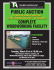More information

### Backup_of_Backup_of_World Mini new page for web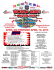More information

### View Auction Flyer - Art Smith AuctioneersMore information

### A family of newforms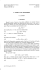More information

### Adapted Toys - UCP of Nebraska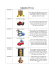More information

### Backdoor Use in Targeted Attacks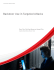More information

### 17) Summa Brasiliensis Mathematicae, II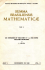More information

### view/download the Archive Media section of our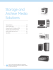More information

### Owner`s Manual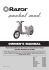More information

### refinement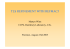More information

### BlackDiamond® 10808 - Smart Technologies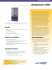More information